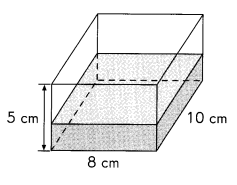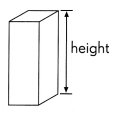# Math in Focus Grade 5 Chapter 15 Answer Key Surface Area and Volume

This handy Math in Focus Grade 5 Workbook Answer Key Chapter 15 Surface Area and Volume provides detailed solutions for the textbook questions.

## Math in Focus Grade 5 Chapter 15 Answer Key Surface Area and Volume

Math Journal

This rectangular container is $$\frac{2}{5}$$-filled with water.
How much more water is needed to increase the height of the water level to 3 centimeters?Show two methods of solving this problem. Which method do you prefer? Why?
Given rectangular tank has volume 8 cm X 10 cm X 5 cm = 400 cm3,
Method 1:
Knowing the volume of tank and water filled in it
as the rectangular container is $$\frac{2}{5}$$-filled with water.
$$\frac{2}{5}$$ X 400 cm3 = 160 cm3, filled with water.
as 1 cubic centimeter is equal to 1 milliliters,

Challenging Practice

Question 1.
A rectangular tank is half-filled with water.
Another 650 cubic centimeters of water are needed to make it $$\frac{3}{5}$$ full.
How much water will be in the tank when it is $$\frac{3}{5}$$ full?
3,900 cubic centimeters of water will be in the tank when it is $$\frac{3}{5}$$ full,

Explanation:
Given a rectangular tank is half-filled with water.
Another 650 cubic centimeters of water are needed to make it $$\frac{3}{5}$$ full.
Let x cubic centimeters of water so $$\frac{1}{2}$$x + $$\frac{3}{5}$$x = 650 cm3 + x,
$$\frac{5x + 6x}{10}$$ = 650 cm3 + x,
$$\frac{11x}{10}$$ = 650 cm3 + x,
11 x = 10 X (650 cm3 + x),
11 x =  6500 cm3 + 10 x,
11x – 10 x = 6,500 cm3,
x = 6,500 cm3,
So, water will be in the tank when it is $$\frac{3}{5}$$ full is
$$\frac{3}{5}$$ x 6,500 cm3 = $$\frac{3 X 6,500}{5}$$ cm3,
$$\frac{19,500}{5}$$ cm3 = 3,900 cm3,
therefore 3,900 cubic centimeters of water will be in the tank when it is $$\frac{3}{5}$$ full.

Question 2.
A cube has a surface area of 21 6 square centimeters.
A second cube has edges that are 3 times as long.
How much greater is the surface area of the second cube than the first cube?
1,728 cm2 greater is the surface area of the second cube than the first cube,

Explanation:
Given a cube has a surface area of 216 square centimeters as
surface area of cube is 6 X a2= 216 cm2,
a2 = 36 cm2 = 6 cm X 6 cm,
edge a    = 6 cm,
A second cube has edges that are 3 times long as
6 cm X 3 = 18 cm, Surface area of second cube is 6 x (18)2 =
6 X 18 cm X 18 cm = 1,944 cm2, Now compairing much greater is the
surface area of the second cube than the first cube is 1,944 cm2 – 216 cm2 = 1,728 cm2.

Problem Solving

A prism has a square base whose edges each measure 5 centimeters.
The ratio of its height to its width is 4 : 1.
Find the volume of the rectangular prism in cubic centimeters.